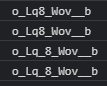# Getting Stuck on CamelCase to underscore

I’m solving `CamelCase to underscore ` challenge on `codewars` site.
I pass all the tests except the random test.
So I need to know what are my mistakes on my code and what I should do to improve my code.
My code so far:

``````const toUnderScore = name => {
return name.replace(/([a-z])([A-Z])|([A-Z])([A-Z])/g, "\$1_\$2").replace(/([A-Z])([A-Z])/g, "\$1_\$2").replace(/([A-Za-z])(\d{1,})/g, "\$1_\$2").replace(/(\d{1,})([A-Z])/g, "\$1_\$2");
}
``````

Link to the challenge: Training on CamelCase to underscore | Codewars

Have you tried debugging your code?

``````const toUnderScore = name => {
let one = name.replace(/([a-z])([A-Z])|([A-Z])([A-Z])/g, "\$1_\$2")
console.log(one)
let two = one.replace(/([A-Z])([A-Z])/g, "\$1_\$2")
console.log(two)
let three = two.replace(/([A-Za-z])(\d{1,})/g, "\$1_\$2")
console.log(three)
let four = three.replace(/(\d{1,})([A-Z])/g, "\$1_\$2");
console.log(four)
return four
}
toUnderScore("oLq8_Wov_ZTb")
``````your first regex is eating away the `ZT` part (because those are actually the `\$3` and `\$4` capture groups, in that case `\$1` and `\$2` are just `''` meaning that `ZT` is substituted with just `_`)
to have only two capture groups total, maybe you need to have the OR inside one of the capture groups

So this code part need a separate `replace method`
Am I correct?

you could fix the regex, you don’t need an even different replace method

This topic was automatically closed 182 days after the last reply. New replies are no longer allowed.